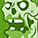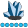## Enable variable attributes to be inherited

I often find myself creating slightly different variables from existing variables. It would be useful to have a facility to enable inheritance of attributes.

For example, I might have a variable named XYZ where missing has been coded as -9, and I need to create a new variable (XYZm) where I am going to convert -9 to . (SAS missing). I'd like to be able to type something like:

XYZm = inherit(XYZ);

if XYZ = -9 then XYZm = . ; ...etc.

This would be particularly useful where the attributes have been set in another program, and copy/paste of code is not straightforward.Super User

You may want to be a bit more detailed about exactly what is going on in this example.

You say you have a variable XYZ "where missing as been coded as -9". Is this done as a numeric assignment, an informat that read . as -9, a format that displays missing values as -9?

I believe that in two of the three cases nothing needs to be done for XYZm = xyz. to have the original missing value of xyy as missing for XYZm.Lapis Lazuli | Level 10

Sorry, my example wasn't very good.

I'm suggesting a function that enables the inheritance of all attributes, particularly the label.

Here is some code that illustrates the problem:

``````title 'Example';

data test;
attrib XYZ length=4 label="Variable of interest";
input ABC XYZ;
cards;
1 0027
2 0039
3 0103
4 -9
5 7890
;

data test2;
set test;
if XYZ = -9 then XYZm = .;
else XYZm = XYZ;
run;

proc contents data=test2;
run;``````

This will produce the following table:It would be helpful if XYZm inherited all of the attributes of XYZ.

Hope that clarifies.Super User

That would be extremely hard to do. What syntax would you propose that SAS could recognize that you wanted this new behavior?  You couldn't modify how SAS handles a simple assignment statement as that would break zillions of lines of existing code that depend on the current behavior.

To copy type/length/format/informat/label just read the variable twice using RENAME= dataset option.

``````data want ;
set have ;
set have (keep=XYZ rename=(XYZ=XYZm));
XYZm = ....;
run;``````Lapis Lazuli | Level 10

I was proposing a new function: inherit

XYZm = inherit(XYZ) ;

However, your solution looks good. I'll try it!